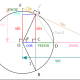# What is i to the power of i?

When you first learnt that$\boxed{i=\sqrt{-1}}$, you have entered the mysterious world of complex numbers.

A mystifying question would be to ask, what is i to the power of i? Is it a complex number?

The surprising answer is that$i^i$ is a real number! Let us explain it here:

The key step is to use Euler’s formula:$\boxed{e^{i\pi}=-1}$. This has been voted as the most beautiful equation in mathematics by many people.

Then,$i=\sqrt{-1}=(-1)^{1/2}={(e^{i\pi})}^{1/2}=e^{i\pi /2}$

Hence,$i^i=e^{i^2\pi /2}=e^{-\pi /2}\approx 0.208$

It is really amazing that an imaginary number to the power of an imaginary number gives a real number, isn’t it? Leave your comments below!## Author: mathtuition88

https://mathtuition88.com/

## 2 thoughts on “What is i^i ?”

1.delarsea says:

Reblogged this on How and Why? and commented:
imaginary powers are interesting things

Like

1.mathtuition88 says:

Thanks for reblogging!

Like

This site uses Akismet to reduce spam. Learn how your comment data is processed.